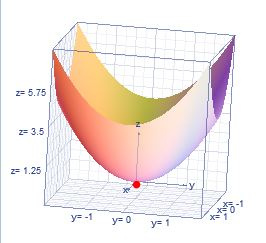# How to check whether that a multi-variable function is convex?

## Question:

How to check whether that a multi-variable function is convex?

## Concavity:

The concavity of a function can be concave up (concave) or concave down (convex), we can show the concavity of a function with the second derivative of the function.

We can know the concavities of a multivariable function with the second derivative.

If the function has a minimum it is concave up.

If the function has a maximum it is convex (concave down).

When the function is multivariable we know the type of critical point (Maximum, Minimum or Saddle point) with the Hessian {eq}D(a,b) \\ {/eq}

If {eq}D(a,b) > 0 \; \text{&} \; f_{xx} > 0 \; \Rightarrow \; \; \text{ f has a relative minimum point at (a,b, f(a,b))} \\ D(a,b) > 0 \; \text{&} \; f_{xx} < 0 \; \Rightarrow \; \; \text{ f has a relative maximum point at (a,b, f(a,b))} \\ D(a,b) < 0 \; \; \Rightarrow \; \; \text{then (a,b, f(a,b)) is a saddle point} \\ D(a,b) = 0 \Rightarrow \; \; \text{ the test is inconclusive} \\ {/eq}

Example:

We have the function

{eq}f(x,y)=x^2+y^2 {/eq}

Graph the functionThe function has a minimum and is convex.